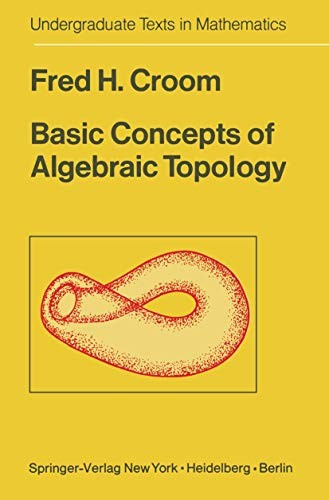• Free Shipping on all orders in Australia
• Over 7 million books in stock
• Proud to be B-Corp
• We aim to be carbon neutral by 2022
• Over 120,000 Trustpilot reviews
Item 1 of 0# Basic Concepts of Algebraic Topology by F.H. Croom

Condition - New
\$102.29
Only 2 left

## Summary

This text is intended as a one semester introduction to algebraic topology at the undergraduate and beginning graduate levels. The prerequisites for this course are calculus at the sophomore level, a one semester introduction to the theory of groups, a one semester introduc tion to point-set topology and some familiarity with vector spaces.

## Basic Concepts of Algebraic Topology Summary

### Basic Concepts of Algebraic Topology by F.H. Croom

This text is intended as a one semester introduction to algebraic topology at the undergraduate and beginning graduate levels. Basically, it covers simplicial homology theory, the fundamental group, covering spaces, the higher homotopy groups and introductory singular homology theory. The text follows a broad historical outline and uses the proofs of the discoverers of the important theorems when this is consistent with the elementary level of the course. This method of presentation is intended to reduce the abstract nature of algebraic topology to a level that is palatable for the beginning student and to provide motivation and cohesion that are often lacking in abstact treatments. The text emphasizes the geometric approach to algebraic topology and attempts to show the importance of topological concepts by applying them to problems of geometry and analysis. The prerequisites for this course are calculus at the sophomore level, a one semester introduction to the theory of groups, a one semester introduc tion to point-set topology and some familiarity with vector spaces. Outlines of the prerequisite material can be found in the appendices at the end of the text. It is suggested that the reader not spend time initially working on the appendices, but rather that he read from the beginning of the text, referring to the appendices as his memory needs refreshing. The text is designed for use by college juniors of normal intelligence and does not require "mathematical maturity" beyond the junior level.

1 Geometric Complexes and Polyhedra.- 1.1 Introduction.- 1.2 Examples.- 1.3 Geometric Complexes and Polyhedra.- 1.4 Orientation of Geometric Complexes.- 2 Simplicial Homology Groups.- 2.1 Chains, Cycles, Boundaries, and Homology Groups.- 2.2 Examples of Homology Groups.- 2.3 The Structure of Homology Groups.- 2.4 The Euler-Poincare Theorem.- 2.5 Pseudomanifolds and the Homology Groups of Sn.- 3 Simplicial Approximation.- 3.1 Introduction.- 3.2 Simplicial Approximation.- 3.3 Induced Homomorphisms on the Homology Groups.- 3.4 The Brouwer Fixed Point Theorem and Related Results.- 4 The Fundamental Group.- 4.1 Introduction.- 4.2 Homotopic Paths and the Fundamental Group.- 4.3 The Covering Homotopy Property for S1.- 4.4 Examples of Fundamental Groups.- 4.5 The Relation Between H1(K) and ?1(|K|).- 5 Covering Spaces.- 5.1 The Definition and Some Examples.- 5.2 Basic Properties of Covering Spaces.- 5.3 Classification of Covering Spaces.- 5.4 Universal Covering Spaces.- 5.5 Applications.- 6 The Higher Homotopy Groups.- 6.1 Introduction.- 6.2 Equivalent Definitions of ?n(X, x0).- 6.3 Basic Properties and Examples.- 6.4 Homotopy Equivalence.- 6.5 Homotopy Groups of Spheres.- 6.6 The Relation Between Hn(K) and ?n(|K|).- 7 Further Developments in Homology.- 7.1 Chain Derivation.- 7.2 The Lefschetz Fixed Point Theorem.- 7.3 Relative Homology Groups.- 7.4 Singular Homology Theory.- 7.5 Axioms for Homology Theory.- Appendix 1. Set Theory.- Appendix 2. Point-set Topology.- Appendix 3. Algebra.

NLS9780387902883
9780387902883
0387902880
Basic Concepts of Algebraic Topology by F.H. Croom
New
Paperback
Springer-Verlag New York Inc.
1978-03-18
180
N/A
Book picture is for illustrative purposes only, actual binding, cover or edition may vary.
This is a new book - be the first to read this copy. With untouched pages and a perfect binding, your brand new copy is ready to be opened for the first time